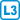## Seven dinners

Peter Generous hosts a dinner for his friends every evening. Three guests are invited to each dinner. In how many ways can Peter send invitations to his 7 friends for an entire week such that each of these seven friends is invited at least once?

• #### Solution

For each dinner Peter may invite one of $$\binom73$$ possible triples, and so for the entire week this gives $$\binom73^7$$ possibilities, though this includes possibilities in which some guest is never invited. If one particular individual is never invited, we can follow the same reasoning with six hosts, so there are $$\binom63^7$$ possibilities. If two friends are never invited, there are $$\binom53^7$$ possibilities, however we can choose this pair in $$\binom72$$ ways. We continue similarly for larger numbers of univited friends.

We now compute the numerical result: $$\binom{7}{3}^7 - 7 \binom{6}{3}^7 + 21 \binom{5}{3}^7 - 35 \binom{4}{3}^7 + 35 \binom{3}{3}^7=35^7-7{\cdot} 20^7+21{\cdot}10^7-35{\cdot}4^7+35\\ =55{,}588,723{,}470$$

There are a total of $$\binom{7}{3}^7 - 7 \binom{6}{3}^7 + 21 \binom{5}{3}^7 - 35 \binom{4}{3}^7 + 35 \binom{3}{3}^7=55{,}588,723{,}470$$ possible ways to arrange the invitations.